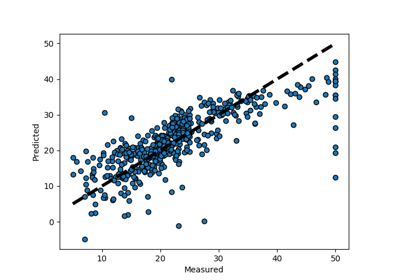/scikit-learn

# sklearn.model_selection.cross_val_predict

`sklearn.model_selection.cross_val_predict(estimator, X, y=None, groups=None, cv=’warn’, n_jobs=None, verbose=0, fit_params=None, pre_dispatch=‘2*n_jobs’, method=’predict’)` [source]

Generate cross-validated estimates for each input data point

It is not appropriate to pass these predictions into an evaluation metric. Use `cross_validate` to measure generalization error.

Read more in the User Guide.

Parameters: `estimator : estimator object implementing ‘fit’ and ‘predict’` The object to use to fit the data. `X : array-like` The data to fit. Can be, for example a list, or an array at least 2d. `y : array-like, optional, default: None` The target variable to try to predict in the case of supervised learning. `groups : array-like, with shape (n_samples,), optional` Group labels for the samples used while splitting the dataset into train/test set. `cv : int, cross-validation generator or an iterable, optional` Determines the cross-validation splitting strategy. Possible inputs for cv are: None, to use the default 3-fold cross validation, integer, to specify the number of folds in a `(Stratified)KFold`, An object to be used as a cross-validation generator. An iterable yielding train, test splits. For integer/None inputs, if the estimator is a classifier and `y` is either binary or multiclass, `StratifiedKFold` is used. In all other cases, `KFold` is used. Refer User Guide for the various cross-validation strategies that can be used here. Changed in version 0.20: `cv` default value if None will change from 3-fold to 5-fold in v0.22. `n_jobs : int or None, optional (default=None)` The number of CPUs to use to do the computation. `None` means 1 unless in a `joblib.parallel_backend` context. `-1` means using all processors. See Glossary for more details. `verbose : integer, optional` The verbosity level. `fit_params : dict, optional` Parameters to pass to the fit method of the estimator. `pre_dispatch : int, or string, optional` Controls the number of jobs that get dispatched during parallel execution. Reducing this number can be useful to avoid an explosion of memory consumption when more jobs get dispatched than CPUs can process. This parameter can be: None, in which case all the jobs are immediately created and spawned. Use this for lightweight and fast-running jobs, to avoid delays due to on-demand spawning of the jobs An int, giving the exact number of total jobs that are spawned A string, giving an expression as a function of n_jobs, as in ‘2*n_jobs’ `method : string, optional, default: ‘predict’` Invokes the passed method name of the passed estimator. For method=’predict_proba’, the columns correspond to the classes in sorted order. `predictions : ndarray` This is the result of calling `method`

`cross_val_score`
calculate score for each CV split
`cross_validate`
calculate one or more scores and timings for each CV split

#### Notes

In the case that one or more classes are absent in a training portion, a default score needs to be assigned to all instances for that class if `method` produces columns per class, as in {‘decision_function’, ‘predict_proba’, ‘predict_log_proba’}. For `predict_proba` this value is 0. In order to ensure finite output, we approximate negative infinity by the minimum finite float value for the dtype in other cases.

#### Examples

```>>> from sklearn import datasets, linear_model
>>> from sklearn.model_selection import cross_val_predict
>>> X = diabetes.data[:150]
>>> y = diabetes.target[:150]
>>> lasso = linear_model.Lasso()
>>> y_pred = cross_val_predict(lasso, X, y, cv=3)
```

## Examples using `sklearn.model_selection.cross_val_predict`Plotting Cross-Validated Predictions